# Centrality dependence of the pseudorapidity density distribution for charged particles in Pb-Pb collisions at $\sqrt{s_{\rm NN}}=5.02$ TeV

We present the charged-particle pseudorapidity density in Pb-Pb collisions at $\sqrt{s_{\mathrm{NN}}}=5.02\,\mathrm{Te\kern-.25exV}$ in centrality classes measured by ALICE. The measurement covers a wide pseudorapidity range from $-3.5$ to $5$, which is sufficient for reliable estimates of the total number of charged particles produced in the collisions. For the most central (0-5%) collisions we find $21\,400\pm 1\,300$ while for the most peripheral (80-90%) we find $230\pm 38$. This corresponds to an increase of $(27\pm4)\%$ over the results at $\sqrt{s_{\mathrm{NN}}}=2.76\,\mathrm{Te\kern-.25exV}$ previously reported by ALICE. The energy dependence of the total number of charged particles produced in heavy-ion collisions is found to obey a modified power-law like behaviour. The charged-particle pseudorapidity density of the most central collisions is compared to model calculations --- none of which fully describes the measured distribution. We also present an estimate of the rapidity density of charged particles. The width of that distribution is found to exhibit a remarkable proportionality to the beam rapidity, independent of the collision energy from the top SPS to LHC energies.

Figures

## Figure 1

 Charged-particle pseudorapidity density for ten centrality classes over a broad $\eta$ range in Pb-Pb collisions at $\sqrt{s_{\rm NN}}=5.02$ TeV. Boxes around the points reflect the total uncorrelated systematic uncertainties, while the filled squares on the right reflect the correlated systematic uncertainty (evaluated at $\eta=0$). Statistical errors are generally insignificant and smaller than the markers. Also shown is the reflection of the $3.5< \eta< 5$ values around $\eta=0$ (open circles). The line corresponds to fits of the difference between two Gaussians centred at $\eta=0$ ($f_{\rm GG}$)  to the data.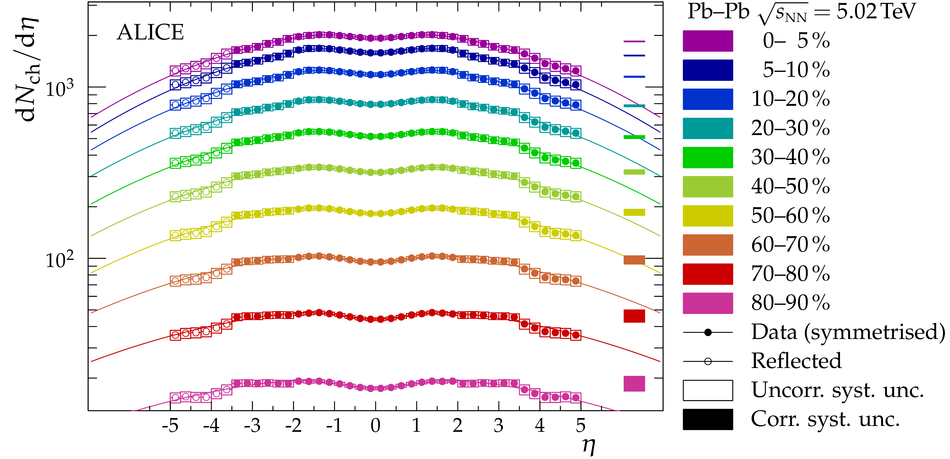## Figure 2

 Total number of charged particles as a function of the mean number of participating nucleons . The total charged-particle multiplicity is given as the integral over d$N_{\rm ch}$/d$\eta$ over the measured region ($-3.5< \eta< 5$) and extrapolations from fitted functions in the unmeasured regions. The contribution from unmeasured $\eta$ regions amounts to $\approx30\%$ of the total number of charged particles. The uncertainty on the extrapolation to the unmeasured pseudorapidity region is smaller than the size of the markers. The contribution to the systematic uncertainties from the centrality determination and electromagnetic processes are vanishing compared to the contribution from the largest differences between the fitted functions. A function inspired by $factorisation$  is fitted to the data, and the best fit yields $a=51.5\pm7.3$,$b=0.16\pm0.05$.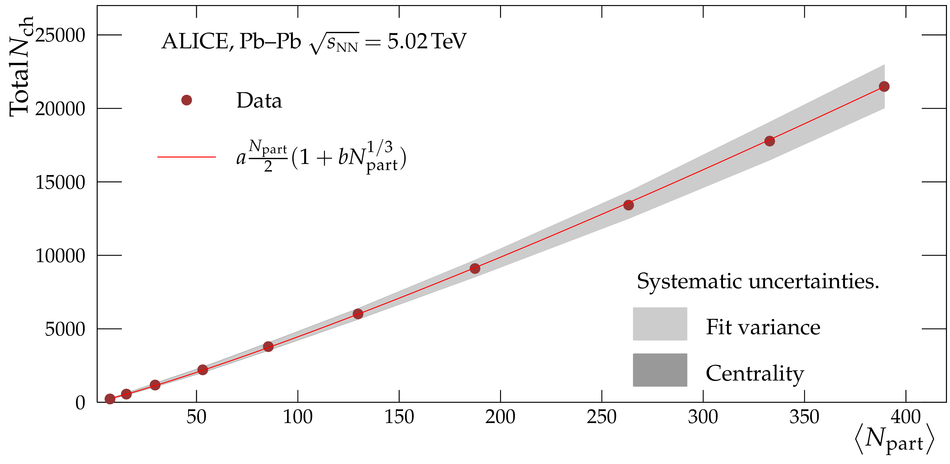## Figure 3

 Comparison of d$N_{\rm ch}$/d$\eta$ in the $0$-$5\%$ ($0$-$6\%$ for KLN) most central collisions of two versions of HIJING, KLN, and EPOS-LHC model calculations to the measured distribution.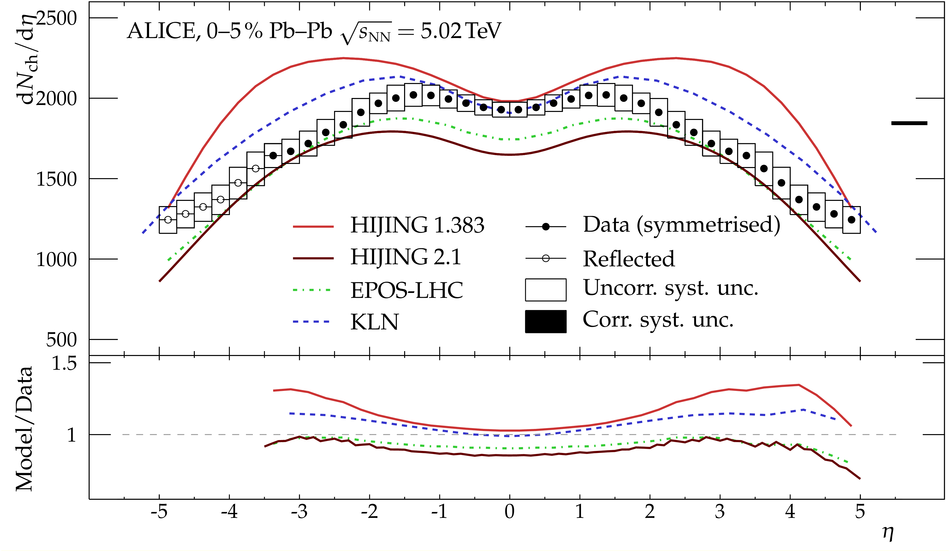## Figure 4

 Total number of charged particles as a function of $\sqrt{s_{\rm NN}}$ for the most central collisions at AGS (0-5% Au-Au) [25,26], SPS (0-5% Pb-Pb) [27,28], RHIC (0-5% and 0-6% Au-Au) [18,29,30] and LHC (0-5% Pb-Pb) . The dotted, dashed, and full lines are extrapolations from fits to lower energy results , while the dash-dotted line is a fit over all energies, including $\sqrt{s_{\rm NN}}=5.02$ TeV.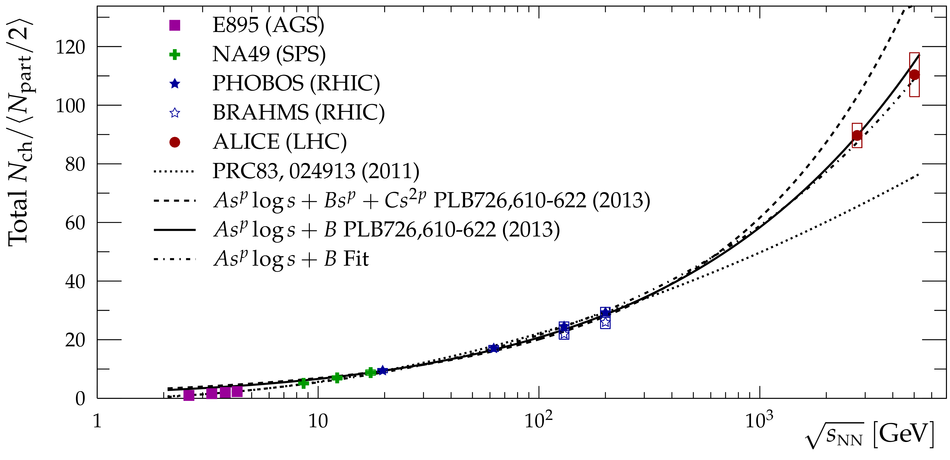## Figure 5

 Estimate of d$N_{\rm ch}$/d$y$ in the most central (0-5%) Pb-Pb collisions at $\sqrt{s_{\rm NN}}=5.02$ TeV. Also shown are the Landau-Wong , Landau-Carruthers , Gaussian, and double-Gaussian distributions.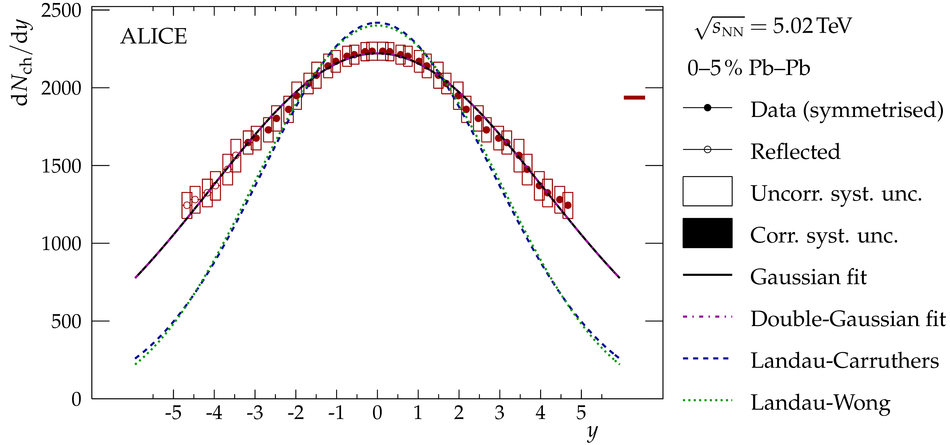## Figure 6

 Scaling behaviour as a function $\sqrt{s_{\rm NN}}$ of the width of the charged-particle or -pion rapidity-density distribution with respect to the Landau-Carruthers width (top) and rapidity range (bottom). Charged-pion points from AGS and SPS are adapted from the literature , while the PHOBOS (filled crosses)  and BRAHMS (open crosses)  charged-hadron points are translated from the corresponding d$N_{\rm ch}$/d$\eta$ results.